In this post, we’ll evaluate and compare the results of several text classification results for the 5-class Stanford Sentiment Treebank (SST-5) dataset.

“Learning to choose is hard. Learning to choose well is harder. And learning to choose well in a world of unlimited possibilities is harder still, perhaps too hard.” — Barry Schwartz

When starting a new NLP sentiment analysis project, it can be quite an overwhelming task to narrow down on a select methodology for a given application. Do we use a rule-based model, or do we train a model on our own data? Should we train a neural network, or will a simple linear model meet our requirements? Should we spend the time and effort in implementing our own text classification framework, or can we just use one off-the-shelf? How hard is it to interpret the results and understand why certain predictions were made?

This series aims at answering some of the above questions, with a focus on fine-grained sentiment analysis. Through the remaining sections, we’ll compare and discuss classification results using several well-known NLP libraries in Python. The methods described below fall under three broad categories:

Rule-based methods:

• TextBlob: Simple rule-based API for sentiment analysis
• VADER: Parsimonious rule-based model for sentiment analysis of social media text.

Feature-based methods:

Embedding-based methods:

• FastText: An NLP library that uses highly efficient CPU-based representations of word embeddings for classification tasks.
• Flair: A PyTorch-based framework for NLP tasks such as sequence tagging and classification.

Each approach is implemented in an object-oriented manner in Python, to ensure that we can easily swap out models for experiments and extend the framework with better, more powerful classifiers in the future.

Why Fine-grained Sentiment?

In most cases today, sentiment classifiers are used for binary classification (just positive or negative sentiment), and for good reason: fine-grained sentiment classification is a significantly more challenging task! The typical breakdown of fine-grained sentiment uses five discrete classes, as shown below. As one might imagine, models very easily err on either side of the strong/weak sentiment intensities thanks to the wonderful subtleties of human language.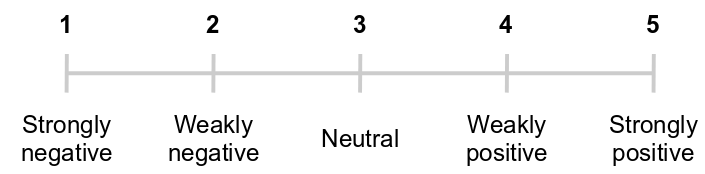Typical class labels (or intensities) for fine-grained sentiment classification

Binary class labels may be sufficient for studying large-scale positive/negative sentiment trends in text data such as Tweets, product reviews or customer feedback, but they do have their limitations. When performing information extraction with comparative expressions, for example: “This OnePlus model X is so much better than Samsung model X.” — a fine-grained analysis can provide more precise results to an automated system that prioritizes addressing customer complaints. In addition, dual-polarity sentences such as “The location was truly disgusting ... but the people there were glorious.” can confuse binary sentiment classifiers, leading to incorrect class predictions.

The above points provide sufficient motivation to tackle this problem!

Stanford Sentiment Treebank

The Stanford Sentiment Treebank (SST-5, or SST-fine-grained) dataset is a suitable benchmark to test our application, since it was designed to help evaluate a model’s ability to understand representations of sentence structure, rather than just looking at individual words in isolation. SST-5 consists of 11,855 sentences extracted from movie reviews with fine-grained sentiment labels [1–5], as well as 215,154 phrases that compose each sentence in the dataset.

The raw data with phrase-based fine-grained sentiment labels is in the form of a tree structure, designed to help train a Recursive Neural Tensor Network (RNTN) from their 2015 paper. The component phrases were constructed by parsing each sentence using the Stanford parser (section 3 in the paper) and creating a recursive tree structure as shown in the below image. A deep neural network was then trained on the tree structure of each sentence to classify the sentiment of each phrase to obtain a cumulative sentiment of the entire sentence.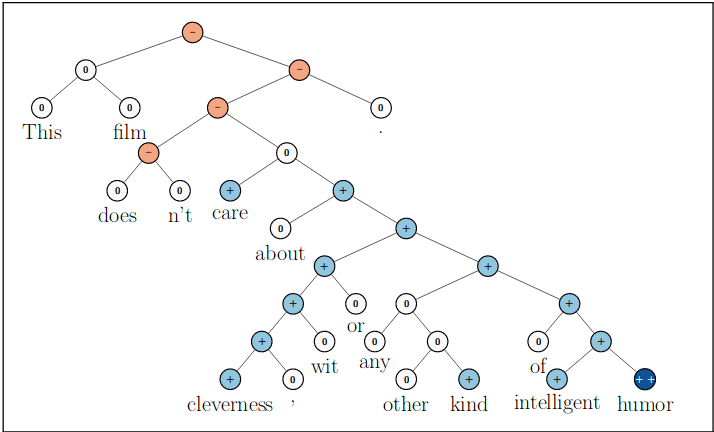Example of Recursive Neural Tensor Network classifying fine-grained sentiment (Source: Original paper)

What is the state-of-the-art?

The original RNTN implemented in the Stanford paper [Socher et al.] obtained an accuracy of 45.7% on the full-sentence sentiment classification. More recently, a Bi-attentive Classification Network (BCN) augmented with ELMo embeddings has been used to achieve a significantly higher accuracy of 54.7% on the SST-5 dataset. The current (as of 2019) state-of-the-art accuracy on the SST-5 dataset is 64.4%, by a method that uses sentence-level embeddings originally designed to solve a paraphrasing task — it ended up doing surprisingly well on fine-grained sentiment analysis as well.

Although neural language models have been getting increasingly powerful since 2018, it might take far bigger deep learning models (with far more parameters) augmented with knowledge-based methods (such as graphs) to achieve sufficient semantic context for accuracies of 70–80% in fine-grained sentiment analysis.

Transform the Dataset to Tabular Form

To evaluate our NLP methods and how each one differs from the other, we will use just the complete samples in the training dataset (ignoring the component phrases since we are not using a recursive tree-based classifier like the Stanford paper). The tree structure of phrases is converted to raw text and its associated class label using the pytreebank library. The code for this tree-to-tabular transformation is provided in this project’s GitHub repo.

The full-sentence text and their class labels (for the train, dev and test sets) are written to individual text files using a tab-delimiter between the sentence and class labels.

Exploratory Data Analysis

We can then explore the tabular dataset in more detail using Pandas. To begin, read in the training set as a DataFrame while specifying the tab-delimiter to distinguish the class label from the text. Note that the class labels in the column “truth” are cast to the data type category in Pandas rather than leaving it as a string.

import pandas as pd
# Read train data
df = pd.read_csv('../data/sst/sst_train.txt', sep='\t', header=None, names=['truth', 'text'])
df['truth'] = df['truth'].str.replace('__label__', '')
df['truth'] = df['truth'].astype(int).astype('category')

Using the command df.shape tells us we have 8,544 training samples.

Is the dataset balanced?

One important aspect to note before analyzing a sentiment classification dataset is the class distribution in the training data.

import matplotlib.pyplot as plt
ax = df[‘truth’].value_counts(sort=False).plot(kind=’barh’)
ax.set_xlabel(“Number of Samples in training Set”)
ax.set_ylabel(“Label”)

It is clear that most of the training samples belong to classes 2 and 4 (the weakly negative/positive classes). A sizeable number of samples belong to the neutral class. Barely 12% of the samples are from the strongly negative class 1, which is something to keep in mind as we evaluate our classifier accuracy.

What about the test set? A quick look tells us that we have 2,210 test samples, with a very similar distribution to the training data — again, there are far fewer samples belonging to the strongly negative/positive classes (1 or 5) compared to the other classes. This is desirable, since the test set distribution on which our classifier makes predictions is not too different from that of the training set.

An interesting point mentioned in the original paper is that many of the really short text examples belong to the neutral class (i.e. class 3). This can be easily visualized in Pandas. We can create a new column that stores the string length of each text sample, and then sort the DataFrame rows in ascending order of their text lengths.

df['len'] = df['text'].str.len()  # Store string length of each sample
df = df.sort_values(['len'], ascending=True)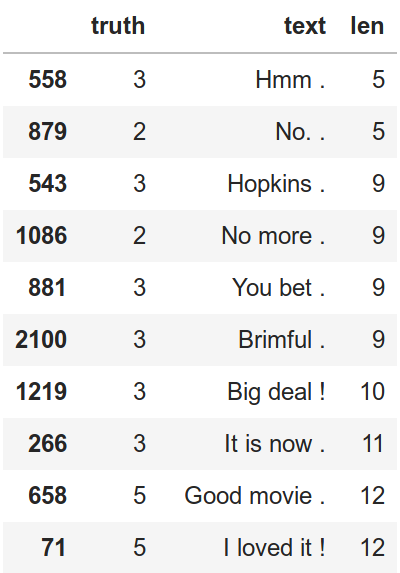Class labels for the really short examples in the test set

Samples with clearly polar words, such as “good” and “loved” would offer greater context to a sentiment classifier— however, for neutral sounding words (such as “Hopkins”, or “Brimful”), the classifier would have to not only work with extremely small context, i.e. single word samples, but also be able to deal with ambiguous or unseen words that did not appear in the training vocabulary.

The data labels aren’t perfect!

As mentioned in the paper, the SST dataset was labelled by human annotators via Amazon Mechanical Turk. Annotators were shown randomly selected phrases for which they chose labels from a continuous slider bar. A discrete sentiment label belonging to one of five classes was reconstructed based on an average of multiple annotators’ chosen labels. Random sampling was used during annotation to ensure that labelling wasn’t influenced by the phrase that preceded it.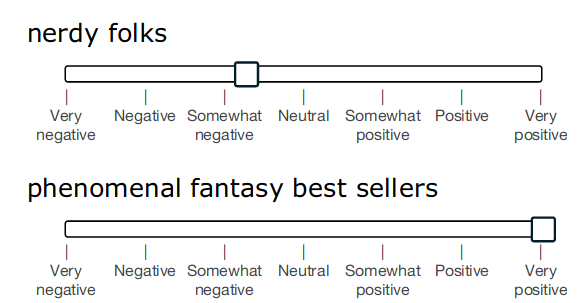Labelling interface for SST dataset (source: Original Paper)

The above example makes it clear why this is such a challenging dataset on which to make sentiment predictions. For example, annotators tended to categorize the phrase “nerdy folks” as somewhat negative, since the word “nerdy” has a somewhat negative connotation in terms of our society’s current perception of nerds. However, from a purely linguistic perspective, this sample could just as well be classified as neutral.

It is thus important to remember that text classification labels are always subject to human perceptions and biases. In a real-world application, it absolutely makes sense to look at certain edge cases on a subjective basis. No benchmark dataset — and by extension, classification model — is ever perfect.

With these points in mind, we can proceed onward to designing our sentiment classification framework!

Methodology

A general workflow for model training and evaluation is shown below.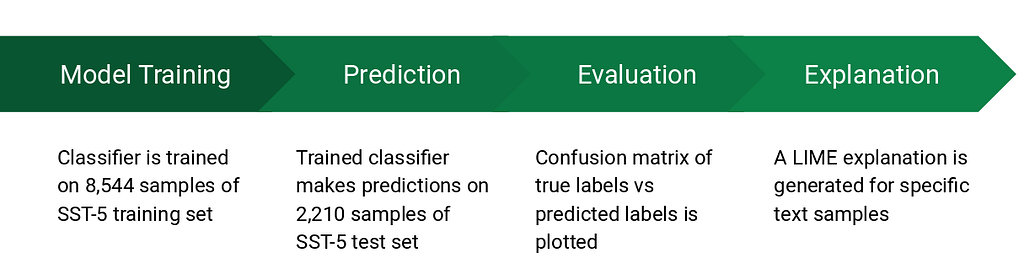Sentiment classification: Training & Evaluation pipeline

Model Training: Each classifier (except for the rule-based ones) is trained on the 8,544 samples from the SST-5 training set using a supervised learning algorithm. Separate training scripts are available in the project’s GitHub repo.

Prediction: As per our object-oriented design philosophy, we avoid repeating code blocks that perform the same tasks across the various classification methods. A Base class is defined in Python that contains the commonly used methods: one for reading in the SST-5 data into a Pandas DataFrame (read_data), and another to calculate the model’s classification accuracy and F1-score (accuracy). Storing the dataset in a Pandas DataFrame this way makes it very convenient to apply custom transformations and user-defined functions while avoiding excessive use of for-loops.

Next, each individual classifier added to our framework must inherit the Base class defined above. To make the framework consistent, a score method and a predict method are included with each new sentiment classifier, as shown below. The score method outputs a unique sentiment class for a text sample, and the predict method applies the score method to every sample in the test dataset to output a new column, 'pred' in the test DataFrame. It is then trivial to compute the model’s accuracy and F1-scores by using the accuracy method defined in the Base class.

Evaluation: To evaluate the model’s accuracy, a confusion matrix of the model is plotted using scikit-learn and matplotlib (plotter.py on GitHub). The confusion matrix tabulates the number of correct predictions versus the number of incorrect predictions for each class, so it becomes easier to see which classes are the least accurately predicted for a given classifier. Note that the confusion matrix for our 5-class case is a normalized anti-diagonal matrix — ideally, the classifier would get almost 100% of its predictions correct so all elements outside the anti-diagonal would be as close to zero as possible.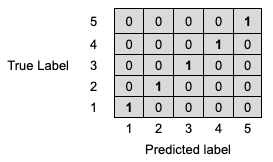Idealized confusion matrix (normalized) — termed an “anti-diagonal matrix”

Training and Model Evaluation

In this section, we’ll go through some key points regarding the training, sentiment scoring and model evaluation for each method.

1 — TextBlob

TextBlob is a popular Python library for processing textual data. It is built on top of NLTK, another popular Natural Language Processing toolbox for Python. TextBlob uses a sentiment lexicon (consisting of predefined words) to assign scores for each word, which are then averaged out using a weighted average to give an overall sentence sentiment score. Three scores: “polarity”, “subjectivity” and “intensity” are calculated for each word.

# A sentiment lexicon can be used to discern objective facts from subjective opinions in text.
# Each word in the lexicon has scores for:
# 1) polarity: negative vs. positive (-1.0 => +1.0)
# 2) subjectivity: objective vs. subjective (+0.0 => +1.0)
# 3) intensity: modifies next word? (x0.5 => x2.0)

Some intuitive rules are hardcoded inside TextBlob to detect modifiers (such as adverbs in English: “very good”) that increase or decrease the overall polarity score of the sentence. A more detailed description of these rules is available in this blog post.

Sentiment Scoring: To convert the polarity score returned by TextBlob (a continuous-valued float in the range [-1, 1]) to a fine-grained class label (an integer), we can make use of binning. This is easily done in Pandas using the pd.cut function — it allows us to go from a continuous variable to a categorical variable by using equal sized bins in the float interval of all TextBlob scores in the results.

Evaluation: Since we are dealing with imbalanced classes during both training and testing, we look at the macro F1 score (which is the harmonic mean of the macro-averaged precision and recall) as well as classification accuracy. As can be seen , the accuracy of the TextBlob classification method is very low, as is the F1 score.

The confusion matrix plot shows more detail about which classes were most incorrectly predicted by the classifier.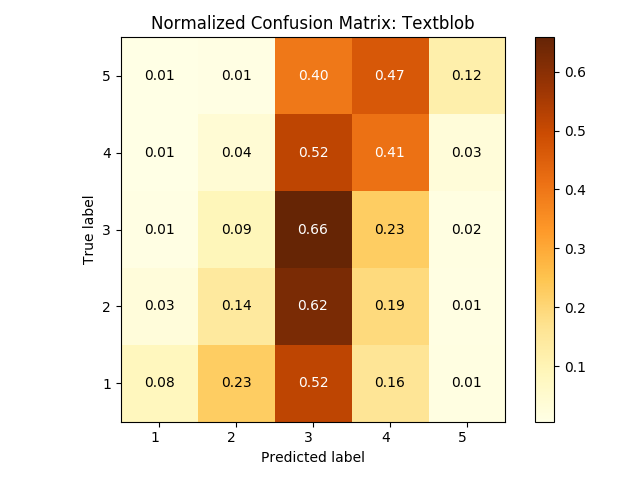Each cell in the confusion matrix shows the percentage of predictions made for the corresponding true label.

To read the above confusion matrix plot, look at the cells along the anti-diagonal. Cell [1, 1] shows the percentage of samples belonging to class 1 that the classifier predicted correctly, cell [2, 2] for correct class 2 predictions, and so on. Cells away from the anti-diagonal show the percentage of wrong predictions made for each respective class — for example, looking at the cell [4, 5], we can see that 47% of all samples that actually belong to class 5 are (incorrectly) predicted as class 4 by TextBlob.

It is clear that our TextBlob classifier predicts most samples as neutral or mildly positive, i.e. of class 3 or 4, which explains why the model accuracy is so low. Very few predictions are strongly negative or positive — this makes sense because TextBlob uses a weighted average sentiment score over all the words in each sample. This can very easily diffuse out the effect of sentences with widely varying polarities between words, such as “This movie is about lying , cheating , but loving the friends you betray.”

Valence Aware Dictionary and sEntiment Reasoner” is another popular rule-based library for sentiment analysis. Like TextBlob, it uses a sentiment lexicon that contains intensity measures for each word based on human-annotated labels. A key difference however, is that VADER was designed with a focus on social media texts. This means that it puts a lot of emphasis on rules that capture the essence of text typically seen on social media — for example, short sentences with emojis, repetitive vocabulary and copious use of punctuation (such as exclamation marks). Below are some examples of the sentiment intensity scores output by VADER.

In the above text samples, minor variations are made to the same sentence. Note that VADER breaks down sentiment intensity scores into a positive, negative and neutral component, which are then normalized and squashed to be within the range [-1, 1] as a “compound” score. As we add more exclamation marks, capitalization and emojis/emoticons, the intensity gets more and more extreme (towards +/- 1).

Sentiment scoring: For returning discrete class values on the SST-5 dataset, we apply a similar technique as done for TextBlob — the continuous “compound” polarity score (float) is converted to a discrete value using binning through the pandas pd.cut function. This returns one of five classes for each test sample, stored as a new column in the resulting DataFrame.

Evaluation: The binning method used above is a rather crude way to equally divide the continuous (float) value from VADER into one of the five discrete classes we require. However, we do see an overall classification accuracy and macro F1 score improvement compared to TextBlob.

The confusion matrix for VADER shows a lot more classes predicted correctly (along the anti-diagonal) — however, the spread of incorrect predictions about the diagonal is also greater, giving us a more “confused” model.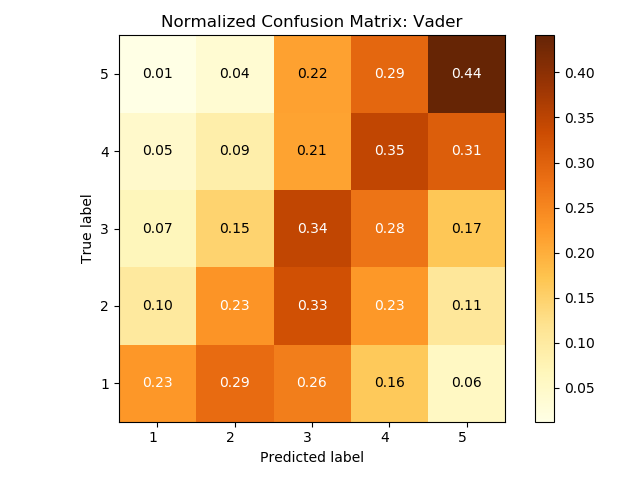Each cell in the confusion matrix shows the percentage of predictions made for the corresponding true label.

The greater spread (outside the anti-diagonal) for VADER can be attributed to the fact that it only ever assigns very low or very high compound scores to text that has a lot of capitalization, punctuation, repetition and emojis. Since SST-5 does not really have such annotated text (it is quite different from social media text), most of the VADER predictions for this dataset lie within the range -0.5 to +0.5 (raw scores). This results in a much more narrow distribution when converting to discrete class labels and hence, many predictions can err on either side of the true label.

Although the result with VADER is still quite low in accuracy, it is clear that its rule-based approach does capture a good amount of fine-gradation in sentiment when compared to TextBlob — fewer cases that are truly negative get classified as positive, and vice versa.

3 — Logistic Regression

Moving onward from rule-based approaches, the next method attempted is a logistic regression — among the most commonly used supervised learning algorithms for classification. Logistic regression is a linear model trained on labelled data — the term linear is important because it means the algorithm only uses linear combinations (i.e. sums and not products) of inputs and parameters to produce a class prediction.

Sebastian Raschka gives a very concise explanation of how the logistic regression equates to a very simple, one-layer neural network in his blog post. The input features and their weights are fed into an activation function (a sigmoid for binary classification, or a softmax for multi-class). The output of the classifier is just the index of the sigmoid/softmax vector with the highest value as the class label.

For multi-class logistic regression, a one-vs-rest method is typically used — in this method, we train C separate binary classification models, where C is the number of classes. Each classifier f_c, for c ∈ {1, …, C} is trained to predict whether a sample is part of class c or not.

Transforming words to features: To transform the text into features, the first step is to use scikit-learn’s CountVectorizer. This converts the entire corpus (i.e. all sentences) of our training data into a matrix of token counts. Tokens (words, punctuation symbols, etc.) are created using NLTK’s tokenizer and commonly-used stop words like “a”, “an”, “the” are removed because they do not add much value to the sentiment scoring. Next, the count matrix is converted to a TF-IDF (Term-frequency Inverse document frequency) representation. From the scikit-learn documentation:

Tf means term-frequency while tf-idf means term-frequency times inverse document-frequency. This is a common term weighting scheme in information retrieval, that has also found good use in document classification. The goal of using tf-idf instead of the raw frequencies of occurrence of a token in a given document is to scale down the impact of tokens that occur very frequently in a given corpus and that are hence empirically less informative than features that occur in a small fraction of the training corpus.

Sentiment scoring: Once we obtain the TF-IDF representation of the training corpus, the classifier is trained by fitting it to the existing features. A “newton-cg” solver is used for optimizing the loss in the logistic regression and L2 regularization is used by default. A sentiment label is returned for each test sample (using scikit-learn’s learner.predict method) as the index of the maximum class probability in the softmax output vector.

Evaluation: Switching from a rule-based method to a feature-based one shows a significant improvement in the overall classification accuracy and F1 scores, as can be seen below.

However, the confusion matrix shows why looking at an overall accuracy measure is not very useful in multi-class problems.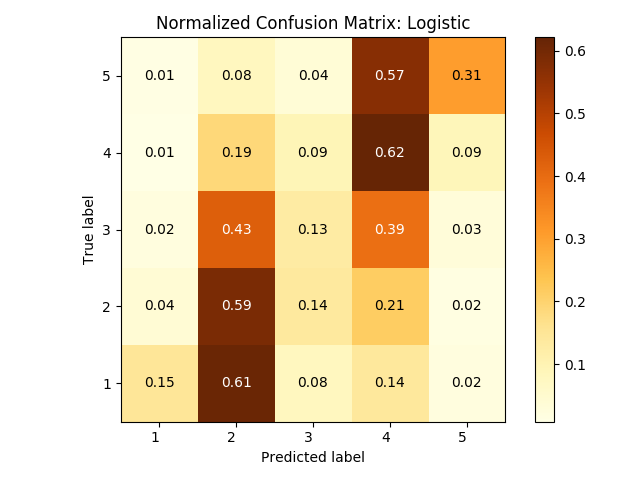Each cell in the confusion matrix shows the percentage of predictions made for the corresponding true label.

The logistic regression model classifies a large percentage of true labels 1 and 5 (strongly negative/positive) as belonging to their neighbour classes (2 and 4). Also, hardly any examples are correctly classified as neutral (class 3). Because most of the training samples belonged to classes 2 and 4, it looks like the logistic classifier mostly learned the features that occur in these majority classes.

4 — Support Vector Machine

Support Vector Machines (SVMs) are very similar to logistic regression in terms of how they optimize a loss function to generate a decision boundary between data points. The primary difference, however, is the use of “kernel functions”, i.e. functions that transform a complex, nonlinear decision space to one that has higher dimensionality, so that an appropriate hyperplane separating the data points can be found. The SVM classifier looks to maximize the distance of each data point from this hyperplane using “support vectors” that characterize each distance as a vector.

A key feature of SVMs is the fact that it uses a hinge loss rather than a logistic loss. This makes it more robust to outliers in the data, since the hinge loss does not diverge as quickly as a logistic loss.

Training and sentiment scoring: The linear SVM in scikit-learn is set up using a similar pipeline as done for the logistic regression described in earlier. Once we obtain the TF-IDF representation of the training corpus, we train the SVM model by fitting it to the training data features. A hinge loss function with a stochastic gradient descent (SGD) optimizer is used, and L2 regularization is applied during training. The sentiment label is returned (using scikit-learn’s learner.predict method) as the index of the maximum class probability in the softmax output vector.

Evaluation: Because quite a few features are likely to be outliers in a realistic dataset, the SVM should in practice produce results that are slightly better than the logistic regression. Looking at the improvement in accuracy and F1 scores, this appears to be true.

The choice of optimizer combined with the SVM’s ability to model a more complex hyperplane separating the samples into their own classes results in a slightly improved confusion matrix when compared with the logistic regression.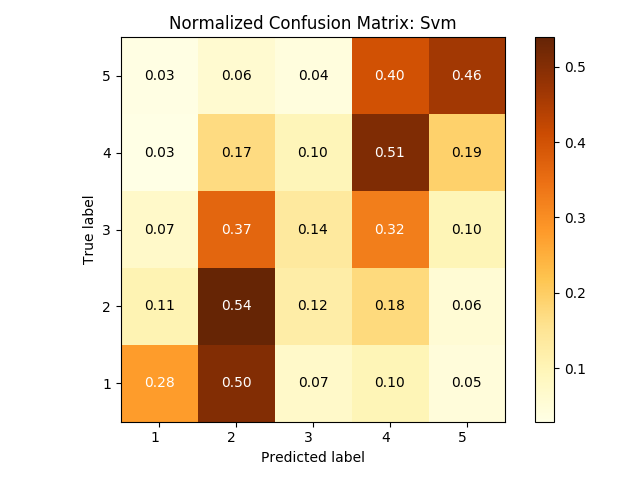Each cell in the confusion matrix shows the percentage of predictions made for the corresponding true label.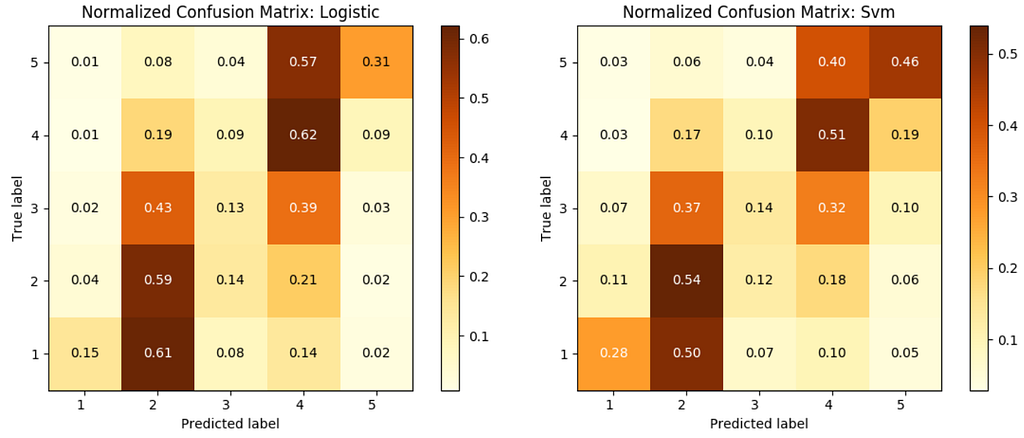Side by side: Logistic Regression vs. SVM

The SVM model predicts the strongly negative/positive classes (1 and 5) more accurately than the logistic regression. However, it still fails to predict enough samples as belonging to class 3— a large percentage of the SVM predictions are once again biased towards the dominant classes 2 and 4. This tells us that there is scope for improvement in the way features are defined. A count vectorizer combined with a TF-IDF transformation does not really learn anything about how words are related to one another — they simply look at the number of word co-occurrences in the each sample to make a conclusion. Enter word embeddings.

5 — FastText

FastText, a highly efficient, scalable, CPU-based library for text representation and classification, was released by the Facebook AI Research (FAIR) team in 2016. A key feature of FastText is the fact that its underlying neural network learns representations, or embeddings that consider similarities between words. While Word2Vec (a word embedding technique released much earlier, in 2013) did something similar, there are some key points that stand out with regard to FastText.

• FastText considers subwords using a collection of n-grams: for example, “train” is broken down into “tra”, “rai” and “ain”. In this manner, the representation of a word is more resistant to misspellings and minor spelling variations.
• Unknown words are handled much better in FastText because it is able to break down long words into subwords that might also appear in other long words, giving it better context.

Python module: Although the source code for FastText is in C++, an official Python module was released by FAIR in June 2019 (after several months of confusion within the community). This makes it very convenient to train and test our model completely within Python, without the use of any external binaries. However, to find the optimum hyperparameters, the command line interface for FastText is recommended.

Training FastText model: To train the FastText model, use the fasttext command line interface (Unix only) — this contains a very useful utility for hyperparameter auto-tuning. As per the documentation, this utility optimizes all hyper-parameters for the maximum F1 score, so we don’t need to do a manual search for the best hyper-parameters for our specific dataset. This is run using the following command on the terminal, and takes about 5 minutes on CPU.

The above command tells FastText to train the model on the training set and validate on the dev set while optimizing the hyper-parameters to achieve the maximum F1-score. The flag -autotune-modelsize 10M tells FastText to optimize the model’s quantization parameters (explained below) such that the final trained model is under 10 MB in size, and the -verbose option is enabled to see which combination of hyper-parameters gives the best results.

💡 TIP: Quantize the FastText model: Quantization reduces the number of bits required to store a model’s weights by using 16 or 8-bit integers, rather than standard 32-bit floating points. Doing so vastly reduces model size (by several orders of magnitude). FastText makes quantization very convenient in the latest release of its command line interface or its Python module as follows (the extension of the quantized model is .ftz, not .bin as the parent model). The cutoff option is set as per the value obtained during hyper-parameter optimization, which ensures that the final model size stays below 10 MB.

# Quantize model to reduce space usage                           model.quantize(input=train, qnorm=True, retrain=True, cutoff=110539)                           model.save_model(os.path.join(model_path, "sst5.ftz"))

The below snippet shows how to train the model from within Python using the optimum hyper-parameters (this step is optional — only the command-line training tool can be used, if preferred).

For more details on the meaning of each hyper-parameter and how FastText works under the hood, this article gives a good description.

Sentiment scoring: Sentiment predictions are made by loading in the trained, quantized (.ftz ) FastText model. The model has a predict method that outputs the most likely labels based on the probabilities extracted from the softmax output layer. For making a class prediction, we simply choose the most likely class label from this list of probabilities, directly extracting it as an integer.

Evaluation: It can be seen that the FastText model accuracy and F1 scores do not vastly improve on the SVM for this dataset.

The F1 score for FastText, however, is slightly higher than that for the SVM.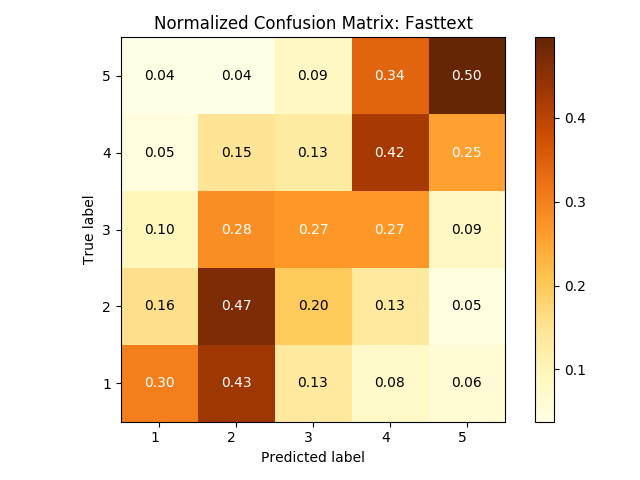Each cell in the confusion matrix shows the percentage of predictions made for the corresponding true label.

The confusion matrix of both models side-by-side highlights this in more detail.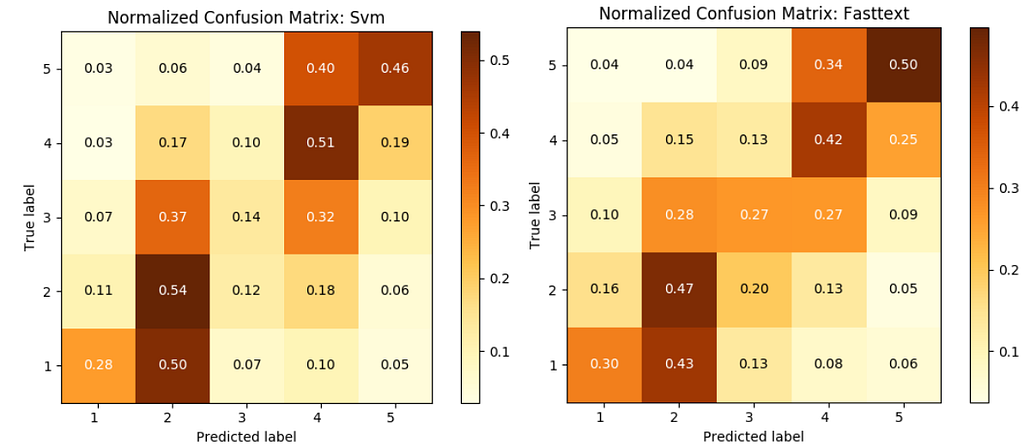Side by side: SVM vs. FastText

The key difference between the FastText and SVM results is the percentage of correct predictions for the neutral class, 3. The SVM predicts more items correctly in the majority classes (2 and 4) than FastText, which highlight the weakness of feature-based approaches in text classification problems with imbalanced classes. Word embeddings and subword representations, as used by FastText, inherently give it additional context. This is especially true when it comes to classifying unknown words, which are quite common in the neutral class (especially the very short samples with one or two words, mostly unseen).

However, our FastText model was trained using word trigrams, so for longer sentences that change polarities midway, the model is bound to “forget” the context several words previously. A sequential model such as an RNN or an LSTM would be able to much better capture longer-term context and model this transitive sentiment.

6 — Flair

In 2018, Zalando Research published a state-of-the-art deep learning sequence tagging NLP library called Flair. This quickly became a popular framework for classification tasks as well because of the fact that it allowed combining different kinds of word embeddings together to give the model even greater contextual awareness.

At the heart of Flair is a contextualized representation called string embeddings. To obtain them, sentences from a large corpus are broken down into character sequences to pre-train a bidirectional language model that “learns” embeddings at the character-level. This way, the model learns to disambiguate case-sensitive characters (for example, proper nouns from similar sounding common nouns) and other syntactic patterns in natural language, which makes it very powerful for tasks like named entity recognition and part-of-speech tagging.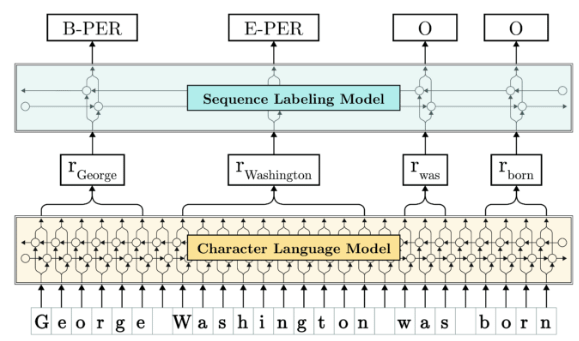Illustration of a BiLSTM sequence labeller with contextual character embeddings (Source)

Training a Flair Model for Classification: What makes Flair extremely convenient yet powerful is its ability to “stack” word embeddings (such as ELMo or BERT) with “Flair” (i.e. string) embeddings. The below example shows how to instantiate a stacked embedding of BERT (base, cased) or ELMo (original) embeddings with Flair embeddings. The stacked representation is converted to a document embedding, i.e. one that gives a single embedding for an entire text sample (no matter how many sentences). This allows us to condense a complex, arbitrary length representation to a fixed-size tensor representation that we can fit in GPU memory for training.

The power of stacking embeddings (either BERT or ELMo) this way comes from the fact that character-level string embeddings capture latent syntactic-semantic information without using the notion of a word (they explicitly focus on subword representations) — while the stacked word embeddings from an external pre-trained neural network model give added word-level context. This enhances the model’s ability to identify a wide range of syntactic features in the given text, allowing it to surpass the performance of classical word embedding models.

Notes on training: The Flair model requires a GPU for training, and due to its LSTM architecture does not parallelize as efficiently as compared to transformer architectures — so training time even on this relatively small SST-5 dataset is of the order of several hours. For this project, 25 epochs of training were run, and the validation loss was still decreasing when training was stopped, meaning that the model was underfitting considerably. As a result, using Flair on a real-world, large dataset for classification tasks can come with a significant cost penalty.

Sentiment scoring: Just as before, a scoring technique is implemented with the existing framework in Pandas. The trained model is first loaded, and the text converted to a Sentence object (which is a tokenized representation of each sentence in a sample). The Flair model’s predict method is called to predict a class label using the maximum index from the softmax output layer, which is then extracted as an integer and stored sample-wise in a Pandas DataFrame. Since model inference can take quite a while even on a GPU, a tqdm progress bar is implemented to show how many test samples the model finished predicting.

Evaluation: Two separate stacked representations are used to train two separate models — one using BERT (base, cased) and the other using ELMo (original). Inference is run using each model to give the following results.

There is a sizeable improvement in accuracy and F1 scores over both the FastText and SVM models! Looking at the confusion matrices for each case yields insights into which classes were better predicted than others.

The above plots highlight why stacking with BERT embeddings scored so much lower than stacking with ELMo embeddings. The BERT case almost makes no correct predictions for class 1 — however it does get a lot more predictions in class 4 correct. The ELMo model seems to stack much better with the Flair embeddings and generates a larger fraction of correct predictions for the minority classes (1 and 5).

What went wrong with the Flair + BERT model during training? It could be that re-projecting and decreasing the number of hidden dimensions (during stacking) resulted in a loss of knowledge from the pre-trained BERT model, explaining why this model did not learn well enough on strongly negative samples. It is not exactly clear why stacking ELMo embeddings results in much better learning compared to stacking with BERT. In both cases, however, the Flair models took a large amount of time (several hours) to train, which can be a huge bottleneck in the real-world —yet, they do highlight the power of using contextual embeddings over classical word embeddings for fine-grained classification.

Conclusions

In this post, six different NLP classifiers in Python were used to make class predictions on the SST-5 fine-grained sentiment dataset. Using progressively more and more complex models, we were able to push up the accuracy and macro-average F1 scores to around 48%, which is not too bad! In a future post, we’ll see how to further improve on these scores using a transformer model powered by transfer learning.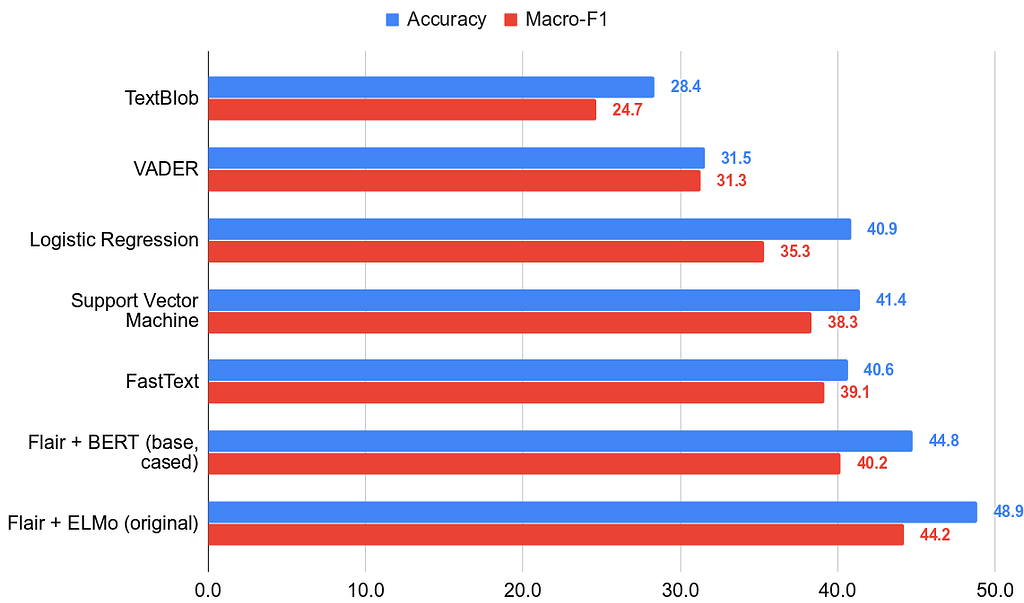Comparison of results: Fine-grained sentiment classification on SST-5

What more can we learn?

Plotting normalized confusion matrices give some useful insights as to why the accuracies for the embedding-based methods are higher than the simpler feature-based methods like logistic regression and SVM. It is clear that overall accuracy is a very poor metric in multi-class problems with a class imbalance, such as this one — which is why macro F1-scores are needed to truly gauge which classifiers perform better.

A key aspect of machine learning models (especially deep learning models) is that they are notoriously hard to interpret. To address this issue, we’ll look at explaining our results and answering the question: “Why did X classifier predict this specific class for this specific sample?”. The LIME Python library is used for this task, which will be described in the next post.

If you made it through to the end of this article, thanks for reading!

• This was Part 1 of a series on fine-grained sentiment analysis in Python.
• Part 2 will cover how to build an explainer module using LIME and explain class predictions on two representative test samples.
• Part 3 (coming soon) will cover how to further improve the accuracy and F1 scores by building our own transformer model and using transfer learning.

NOTE: All the training and evaluation code for this analysis are available in the project’s Github repo, so feel free to reproduce the results and make your own findings!